## 7.8Resolving the viscous sub-layer

Wall functions were introduced in Sec. 7.5 to avoid the need for a large mesh with cells small enough to resolve the boundary layer into the viscous sub-layer. They provide a reasonable prediction of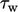using the log law for the velocity distribution in the inertial sub-layer.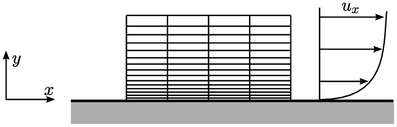A CFD simulation may alternatively use a mesh with suﬃciently thin cells to resolve the ﬂow through the viscous sub-layer, e.g. with near-wall cell centre height corresponding to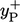= 1, for a more accurate prediction of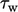. If so, the turbulence model must then be able to function reliably in viscous ﬂow regions.

Such models are usually described as “low Reynolds number”. The expression does not refer to the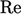of the ﬂow based on the characteristic scales of the problem, e.g. axial mean ﬂow speed and diameter for a pipe. Instead it is a “turbulence” Reynolds number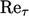based on the scales of speed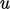and sizeof turbulent eddies and can be deﬁned as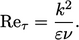(7.27)
This deﬁnition is obtained from scale arguments introduced in Sec. 6.6 , in which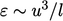. Sincerepresents ﬂuctuations,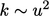. Combining these expressions into a Reynolds number yields Eq. (7.27 ).

### Asymptotic consistency

Low-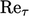turbulence models pay attention to the behaviour of ﬂuctuating velocities, e.g.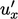,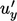, in the limit that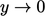at the solid boundary.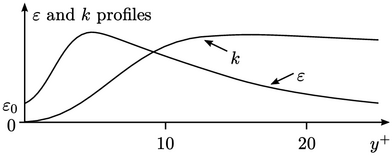They aim to capture the shape the proﬁles of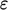and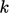as they approach. Let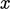and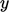deﬁne the directions tangential and normal to the wall respectively. Proﬁles in the ﬂuctuating velocities can be expressed by polynomials in, i.e.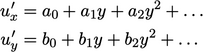where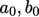, etc. are functions of space and time. The no slip condition implies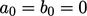, so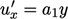to the lowest order in. For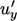, it is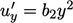since, at the wall,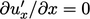and by continuity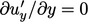.

The turbulent properties are, to the lowest order in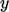, as follows.

• From Sec. 6.11 ,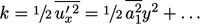• From Eq. (6.30 ),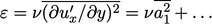It follows that models achieve asymptotic consistency when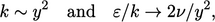(7.28)
in the limit that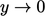.
Notes on CFD: General Principles - 7.8 Resolving the viscous sub-layer